Importance: High ✭✭✭
 Author(s): Alon, Noga Tarsi, Michael
 Subject: Combinatorics
 Keywords: latin square
 Posted by: mdevos on: October 7th, 2007

A latin square is even if the product of the signs of all of the row and column permutations is 1 and is odd otherwise.

Conjecture   For every positive even integer, the number of even latin squares of orderand the number of odd latin squares of orderare different.

For every positive integer, let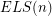, () be the number of even (odd) latin squares of order.

The inspiration for this conjecture comes from an attempt by Alon and Tarsi to use their polynomial technique to show that the complete bipartite graph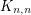is-edge-choosable (a famous conjecture of Dinitz asserts that this is always true). They show (in [AT]) that whenever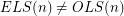, the graphis-edge-choosable. For odd integers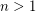it is easy to see that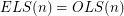, since interchanging the first two rows has no effect on the signs of the rows, but flips the signs of all of the columns. For even, Alon and Tarsi checked thatandwere different forand conjectured that this pattern would continue. Although Dinitz' Conjecture has since been resolved, Alon and Tarsi's conjecture remains quite interesting. In particular, it has been shown by Huang and Rota [HR] that the truth of this conjecture would imply Rota's basis conjecture for even values of(see [O] for a nice proof of this).

ELS() and OLS() appear in the The Encyclopedia of Integer Sequences as A114628 and A114629. The following chart shows the first few values. Although the data here is quite limited,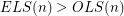for every evenin the chart, and as far as we know, this might hold in general.

 n ELS(n) OLS(n) 1 1 0 2 2 0 3 6 6 4 576 0 5 80640 80640 6 505958400 306892800 7 30739709952000 30739709952000 8 55019078005712486400 53756954453370470400

Drisko [D1] proved that wheneveris prime,(mod), thus verifying the Alon-Tarsi conjecture for any even number which is one more than a prime. Shortly afterward, Zappa [Z] introduced a function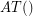which compares the number of even and odd latin squares which have all diagonal entries equal to one, and proved some interesting identities concerning. By utilizing these identities, Drisko [D2] proved thatwheneveris of the formfor a prime.

## Bibliography

*[AT] N. Alon, M. Tarsi, Coloring and Orientations of Graphs. Combinatorica 12, 125-143, 1992 MathSciNet

[D1] A. Drisko, On the number of even and odd Latin squares of order, Adv. Math. 128 (1997), no. 1, 20--35. MathSciNet

[D2] A. Drisko, Proof of the Alon-Tarsi conjecture for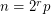. Electron. J. Combin. 5 (1998) MathSciNet.

[HR] R. Huang and G-C Rota, On the relations of various conjectures on Latin squares and straightening coefficients. Discrete Math. 128 (1994), no. 1-3, 225--236. MathSciNet.

[O] S. Onn, A colorful determinantal identity, a conjecture of Rota, and Latin squares. Amer. Math. Monthly 104 (1997), no. 2, 156--159. MathSciNet.

[Z] P. Zappa, The Cayley determinant of the determinant tensor and the Alon-Tarsi conjecture. Adv. in Appl. Math. 19 (1997), no. 1, 31--44. MathSciNet.

* indicates original appearance(s) of problem.